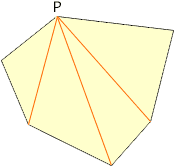I am a student in the 9th grade geometry, I have an example question to study for midterms and I was wondering if you could help me. The question is What is the sum of the measures (interior angles) in an octagon ... heptagon ... decagon. Any help I could get on this is greatly appreciated. Thank you. Hi, I am going to assume that each of the interior angles in the polygons you are considering is less than 180o. Consider the hexagon below.Choose one of the verticies, call it P, and draw the three diagonals. This partitions the hexagon into four triangles. From the diagram the sum of the interior angles of the hexagon is the same as the sum of the interior angles of the four triangles. But the sum of the interior angles of a triangle is 180o and hence the sum of the interior angles of the hexagon is 4x180o = 720o. Using this idea you should be able to find the sum of the interior angles of an octagon...heptagon...decagon... and maybe even find a general expression that works for all of them. Cheers, Penny Go to Math Central